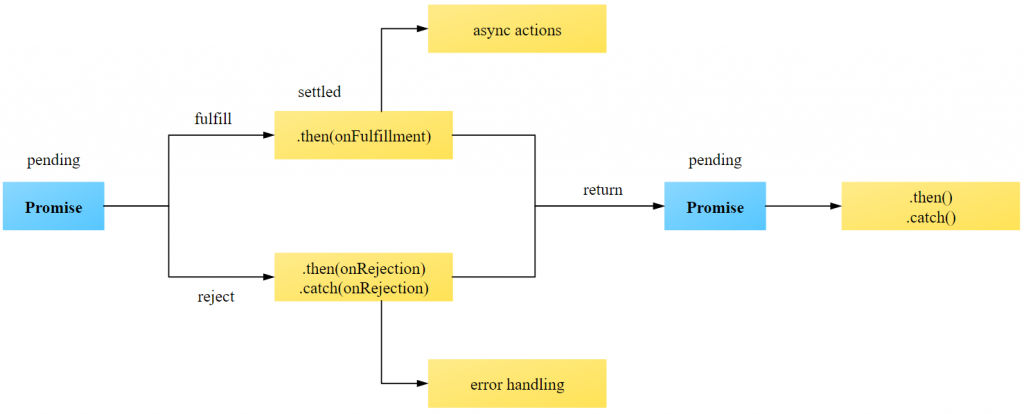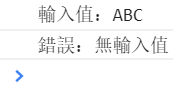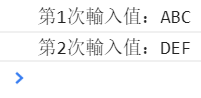#2019 iT 邦幫忙鐵人賽DAY 28
0
Modern Web

## Promise

``````(function misA() {
setTimeout(() => {
console.log('任務A執行完畢');
}, Math.floor(Math.random() * 5000));
})();
(function misB() {
setTimeout(() => {
console.log('任務B執行完畢');
}, Math.floor(Math.random() * 5000));
})();
(function misC() {
setTimeout(() => {
console.log('任務C執行完畢');
}, Math.floor(Math.random() * 5000));
})();
(function misD() {
setTimeout(() => {
console.log('任務D執行完畢');
}, Math.floor(Math.random() * 5000));
})();
``````

``````function misA(callback) {
setTimeout(() => {
console.log('任務A執行完畢');
callback();
}, Math.floor(Math.random() * 2000));
};
function misB(callback) {
setTimeout(() => {
console.log('任務B執行完畢');
callback();
}, Math.floor(Math.random() * 2000));
};
function misC(callback) {
setTimeout(() => {
console.log('任務C執行完畢');
callback();
}, Math.floor(Math.random() * 2000));
};
function misD() {
setTimeout(() => {
console.log('任務D執行完畢');
}, Math.floor(Math.random() * 2000));
};
misA(() => {
misB(() => {
misC(() => {
misD();
});
});
});
``````

#### promise• 擱置（pending）：初始狀態，不是 fulfilled 與 rejected。
• 實現（fulfilled）：表示操作成功地完成。
• 拒絕（rejected）：表示操作失敗了。

promise物件一開始是等待狀態（pending），只有兩種執行結果，不是成功（fulfilled）就是失敗（rejected）。

Promise建構子的語法結構：

``````new Promise((resolve, reject) => {
statement
})
``````

resolve：執行成功時要呼叫的函式。
reject：執行失敗時要呼叫的函式。
statement：程式主體，非同步處理的部分。

``````function asyncFunc(value) {
return new Promise(function (resolve, reject) {
if (value)
resolve(value);
else
reject(reason);
});
}
``````

``````p.then(onFulfilled, onRejected);
p.then((response) => {
// 執行成功
}, (error) => {
// 執行失敗
});
``````

then方法，有2個參數可用：

• onFulfilled是當promise物件執行成功，狀態為實現（fulfilled）呼叫的函式，並有一個傳入值(value)做後續的運算。
• onRejected是當promise物件執行失敗，狀態為拒絕（rejected）呼叫的函式，一樣有一個傳入值(reason)做後續的處理。

then函式會回傳一個新的promise物件，這樣做的目的是能夠使用方法串接（method chaining），讓多個非同步運算，可以一直串接下去。

``````function async (value) {
return new Promise((resolve, reject) => {
setTimeout(() => {
if (value)
resolve(value);
else
reject('無輸入值');
}, 500);
})
}
async ('ABC').then(
response => {
console.log(`輸入值：\${response}`);
}, error => {
console.log(`錯誤：\${error}`);
}
);
async ().then(
response => {
console.log(`輸入值：\${response}`);
}, error => {
console.log(`錯誤：\${error}`);
}
);
````````````async ('ABC').then(
response => {
console.log(`第1次輸入值：\${response}`);
return async ('DEF');
}, error => {
console.log(`錯誤：\${error}`);
}
).then(
response => {
console.log(`第2次輸入值：\${response}`);
}, error => {
console.log(`錯誤：\${error}`);
}
);
``````then函式的2個參數，分別處理實現（fulfilled）與拒絕（rejected）狀態，不過為了方便方法串接（method chaining），通常我們只在then函式，傳入第一個處理實現（fulfilled）的函式參數。

JavaScript Note31